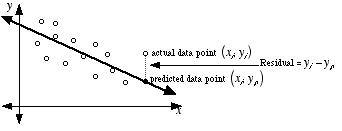index: click on a letter A B C D E F G H I J K L M N O P Q R S T U V W X Y Z A to Z index index: subject areas numbers & symbols sets, logic, proofs geometry algebra trigonometry advanced algebra & pre-calculus calculus advanced topics probability & statistics real world applications multimedia entrieswww.mathwords.com about mathwords website feedback

 Residual The vertical distance between a data point and the graph of a regression equation. The residual is positive if the data point is above the graph. The residual is negative if the data point is below the graph. The residual is 0 only when the graph passes through the data point.See also